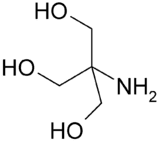# Determining pH when given volume and concentration of a solution?

• Violagirl

## Homework Statement

I'm stuck on these two problems:

A) IF you make a 50 mL of a 0.1 M Tris solution (in acid form), what will be the pH?

B) If you add 2 mL of 1 M NaOH to the above solution, what will be the pH?

## Homework Equations

pH = pka + log [A/HA] Hendersen-Hasselbalch

## The Attempt at a Solution

I'm not sure if HH is the right equation to use or not for this one when given both volume and concentration or if you add the NaOH to it. Help is greatly appreciated.

## Homework Statement

I'm stuck on these two problems:

A) IF you make a 50 mL of a 0.1 M Tris solution (in acid form), what will be the pH?

B) If you add 2 mL of 1 M NaOH to the above solution, what will be the pH?

## Homework Equations

pH = pka + log [A/HA] Hendersen-Hasselbalch

## The Attempt at a Solution

I'm not sure if HH is the right equation to use or not for this one when given both volume and concentration or if you add the NaOH to it. Help is greatly appreciated.

It would help if you could define what "Tris solution (in acid form)" is.

I'm not sure, that is the exact way that the question was asked...Is it referring to a Tris buffer of a 100% solution?

I can only repeat after Pranav-Arora - what does it mean "Tris in acid form"? What other forms can Tris have?